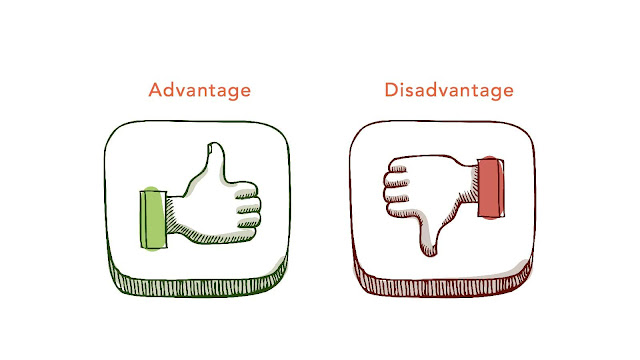# HSSlive: Plus One & Plus Two Notes & Solutions for Kerala State Board

## Thursday, January 26, 2023advantages and disadvantages of 1's complement

## What is 1's complement?

1's complement is a method of representing negative binary numbers. It is used in computers and other digital systems to perform arithmetic operations on signed binary numbers.

In 1's complement, the positive numbers are represented in the same way as in unsigned binary numbers. To represent negative numbers, 1's complement is used. It is obtained by inverting or flipping all the bits of a binary number.

For example, if a binary number is "0110", its 1's complement will be "1001".

To obtain the negative of a binary number, the 1's complement is added to 1. So, the negative of "0110" will be "1001" + 1 = "1010".

1's complement is a simple and efficient method for representing negative binary numbers, but it has some limitations. One of the major limitations is that there is no unique representation for zero. It also requires additional hardware and complexity in the computer to handle the overflow and underflow conditions.

1's complement is a widely used method for representing negative binary numbers in digital systems. It has several advantages over other methods of representing negative numbers, such as 2's complement.

One of the main advantages of 1's complement is its simplicity. It is easy to understand and implement, and it requires minimal hardware. The representation of positive and negative numbers is straightforward, and it is easy to convert between the two.

Another advantage is that 1's complement is easy to perform arithmetic operations on. Addition and subtraction can be performed using the same hardware and logic as for unsigned binary numbers. This makes it a more efficient method of representing negative numbers.

1's complement also has the advantage of making it easy to detect overflow and underflow conditions. In 1's complement, overflow occurs when the result is not representable using the same number of bits as the operands. This is easy to detect by comparing the sign bit of the result with the sign bit of the operands.

One more advantage of 1's complement is that it allows for more efficient error detection and correction in digital systems. Because the most significant bit is reserved for the sign, it can be used as a parity bit to detect errors in the data.

In conclusion, 1's complement is a simple, efficient and easy to understand method of representing negative numbers in digital systems. It has the advantages of simplicity, ease of arithmetic operations, easy overflow and underflow detection and efficient error detection and correction.

1's complement is a widely used method for representing negative binary numbers in digital systems, but it also has several disadvantages.

One major disadvantage is that 1's complement is not a true two's complement representation. In the two's complement system, negative numbers are represented by the two's complement of the absolute value, which makes for a more efficient and straightforward arithmetic. However, 1's complement does not have this feature, which can lead to confusion and errors in calculations.

Another disadvantage is that 1's complement requires more bits to represent the same range of numbers compared to the two's complement system. This is because in 1's complement, the most significant bit is used to indicate the sign of the number, while in two's complement, the most significant bit is used to represent the magnitude of the number. This means that for a given range of numbers, 1's complement requires more bits than two's complement.

Another disadvantage of 1's complement is that it is not as efficient as two's complement in terms of detecting overflow and underflow conditions. In 1's complement, overflow occurs when the result is not representable using the same number of bits as the operands. This is easy to detect by comparing the sign bit of the result with the sign bit of the operands. But, in two's complement, it is much simpler to detect overflow by checking if the carry-out bit is set.

1's complement also has the disadvantage of not having a representation of zero. In 1's complement, the positive and negative numbers are symmetric about zero, so there is no separate representation for zero. This can lead to confusion and errors in calculations.

In conclusion, 1's complement is a widely used method of representing negative binary numbers in digital systems, but it also has several disadvantages. It is not a true two's complement representation, requires more bits to represent the same range of numbers, less efficient in detecting overflow and underflow conditions and doesn't have a representation of zero. These disadvantages make it less preferred than the two's complement system in modern digital systems.

Share: